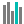#BINOM.DIST (PQL - xl)

This function calculates the individual term binomial distribution probability

• Library: PQL \ Spreadsheet \ Statistical
• Compatibility: Any content (regardless of data source) in the Tabulate spreadsheet module
• Solve: This function can be used with the Solve plug-in: both as part of the objective function and constraint functions

#### Syntax

BINOM.DIST(number s, trials, probability s, cumulative)

##### Function Arguments
 Name Description Type Optional number s Number representing the number of successes in trials; if not an integer, the number is truncated Number trials Number representing the number of independent trials; if not an integer, the number is truncated Number probability s Probability of success on each trial; number between 0 and 1 Number cumulative Logical value that determines the form of the function; if TRUE, then this function returns the cumulative distribution function, which is the probability that there are at most x successes; if FALSE, it returns the probability mass function, which is the probability that there are x successes StringOrNumber